Related Articles

# Class 9 RD Sharma Solutions – Chapter 15 Areas of Parallelograms and Triangles- Exercise 15.3 | Set 1

• Last Updated : 19 Apr, 2021

### Question 1. In the figure, compute the area of quadrilateral ABCD.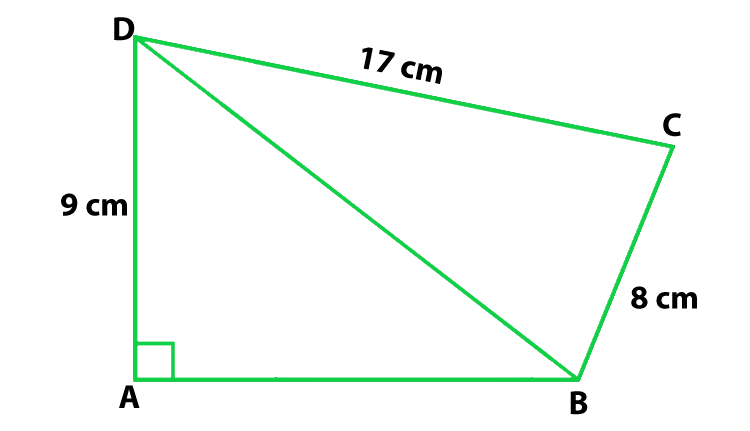Solution:

According to the question

DC = 17 cm, AD = 9 cm and BC = 8 cm

Find: the area of quadrilateral ABCD

In ΔBCD,

Using Pythagoras Theorem

CD2 = BD2 + BC2

172 = BD2 + 82

BD2 = 289 − 64

BD = 15

So, the area of ΔBCD = 1/2(8 × 17) = 68

In ΔABD

Using Pythagoras Theorem

152 = AB2 + 92

AB2 = 225 − 81 = 144

AB = 12

So, the area of ΔABD = 1/2(12 × 9) = 54

Now we find the ar(quad ABCD) = ar(ΔABD) + ar(ΔBCD)

ar(quad ABCD) = 54 + 68 = 122 cm2

Hence, the area of quadrilateral ABCD is 122 cm2

### Question 2. In the figure, PQRS is a square and T and U are, respectively, the midpoints of PS and QR. Find the area of ΔOTS if PQ = 8 cm.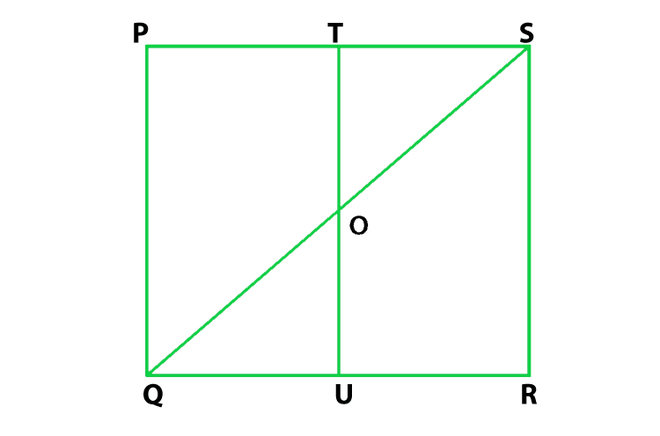Solution:

According to the question

T and U are mid-points of PS and QR

Hence, TU ∥ PQ

PQ = 8 cm

Find: the area of ΔOTS

In ΔPQS,

It is given that T is the midpoint of PS and TO ∥ PQ

therefore, TO = (1/2) PQ = 4 cm

and TS = (1/2) PS = 4 cm

So, the area of ar(ΔOTS) = (1/2)(TO × TS)

= (1/2)(4 × 4) = 8 cm2

Hence, the area of ΔOTS is 8 cm2

### Question 3. Compute the area of trapezium PQRS in figure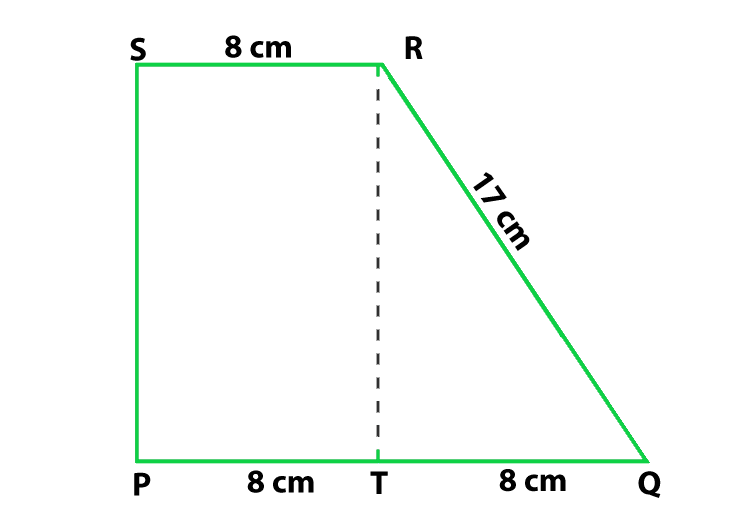Solution:

According to the figure

PQ = 16 cm

Here T is the mid-point of side PQ so

PT = QT = 8 cm

SR = 8 cm

RQ = 17 cm

Find: the area of trapezium PQRS

So, the ar(trap. PQRS) = ar(rect. PSRT) + ar(ΔQRT)  ….(1)

So, In ΔQRT

Using Pythagoras Theorem

QR2 = QT2 + RT2

RT2 = QR2 − QT2

RT2 = 172 − 82 = 225

RT = 15

So, the area of ΔQRT

= 1/2(QT × RT) = 8 × 15 = 180/2 = 60

Now we find the area of rectangle PSRT

= PT × RT = 8 × 15 = 120

Now put all these values in eq(1), we get

ar(trap. PQRS) = 120 + 60 = 180

Hence, the area of trapezium is 180 cm2

### Question 4. In figure, ∠AOB = 90°, AC = BC, OA = 12 cm and OC = 6.5 cm. Find the area of ΔAOB.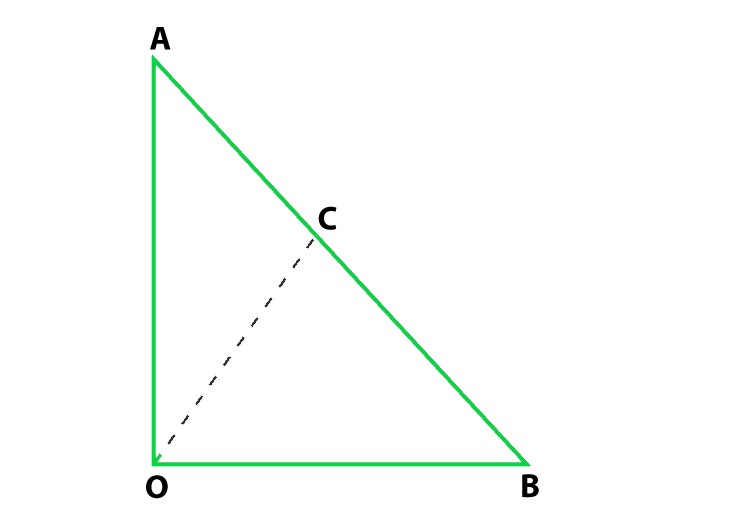Solution:

According to the question

∠AOB = 90°, AC = BC, OA = 12 cm and OC = 6.5 cm

Find: the area of ΔAOB

From the figure, C is the mid-point of hypotenuse AB so in right triangle

the mid-point is equidistant from the vertices

therefore, CA = CB = OC

CA = CB = 6.5 cm

AB = 13 cm

In ΔOAB,

Using Pythagoras Theorem

AB2 = OB2 + OA2

132 = OB2 + 122

OB2 = 132 − 122 = 169 − 144 = 25

OB = 5

Now we find the area of ΔAOB = (1/2)(12 × 5) = 30 cm2

Hence, the area of ΔAOB is 30 cm2

### Question 5. In figure, ABCD is a trapezium in which AB = 7 cm, AD = BC = 5 cm, DC = x cm, and distance between AB and DC is 4 cm. Find the value of x and area of trapezium ABCD.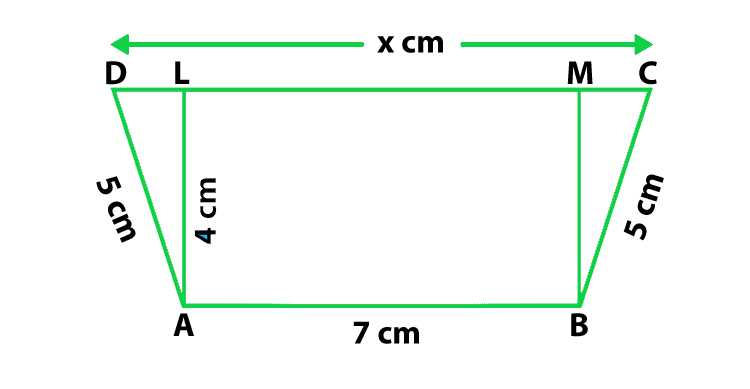Solution:

According to the question

AB = 7 cm, AD = BC = 5 cm

Construction: Draw AL ⊥ DC, BM ⊥ DC,

AL = BM = 4 cm and LM = 7 cm

Find: the value of x and area of trapezium ABCD.

Using Pythagoras Theorem

25 = 16 + DL2

DL = 3 cm

Similarly,

MC = √BC2 – BM2 = √25 – 16 = 3 cm

So, x = CD = CM + ML + LD = (3 + 7 + 3) cm = 13 cm

Now we find the area of trapezium ABCD = 1/2(AB + CD) × AL

= 1/2(7 + 13) × 4 cm2 = 40 cm2

Hence, the value of x is 3 cm and the area of trapezium is 40 cm2

### Question 6. In figure, OCDE is a rectangle inscribed in a quadrant of a circle of radius 10 cm. If OE = 2√5 cm, find the area of the rectangle.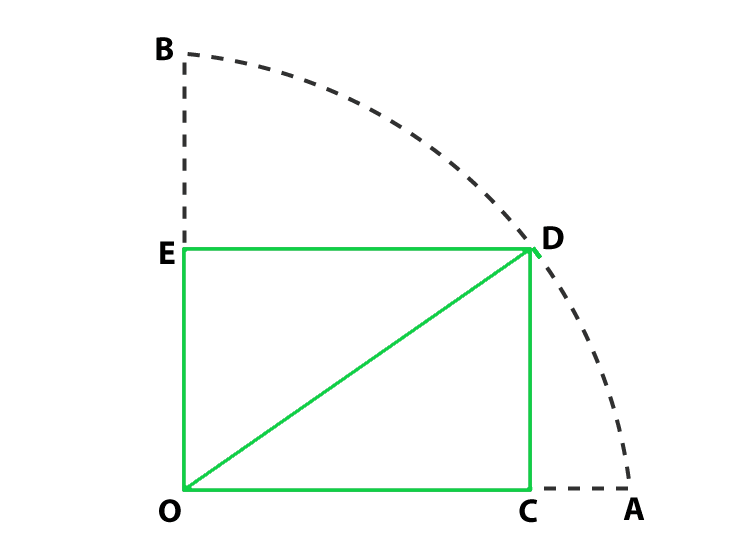Solution:

According to the question

OD = 10 cm and OE = 2√5cm

Find: the area of the rectangle OCDE

So, In ΔDEO

By using Pythagoras theorem

OD2 = OE2 + DE2

DE = √OD2 – OE2

= √102 – (2√5)2 = 4√5 cm

Now we find the area of rectangle OCDE = OE × DE

= 2√5 x 4√5 cm2 = 40 cm2

Hence, the area of rectangle is 40 cm2

### Question 7. In figure, ABCD is a trapezium in which AB ∥ DC. Prove that ar(ΔAOD) = ar(ΔBOC)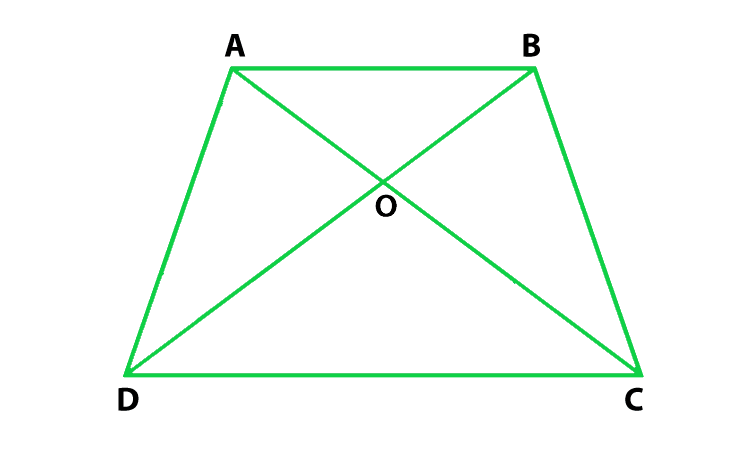Solution:

According to the question

ABCD is a trapezium in which AB ∥ DC

To prove : ar(ΔAOD) = ar(ΔBOC)

Proof :

According to the figure, we conclude that ΔADC and ΔBDC are on the

same base DC and between same parallels AB and DC

Here, ar(ΔADC) = ar(ΔAOD) + ar(ΔDOC)

Similarly, ar(ΔBDC) = ar(ΔBOC) + ar(ΔDOC)

Now put all these values in eq(1), we get

ar(ΔAOD) + ar(ΔDOC) = ar(ΔBOC) + ar(ΔDOC)

ar(ΔAOD) = ar(ΔBOC)

Hence, proved

### Question 8. In figure, ABCD, ABFE and CDEF are parallelograms. Prove that ar(ΔADE) = ar(ΔBCF).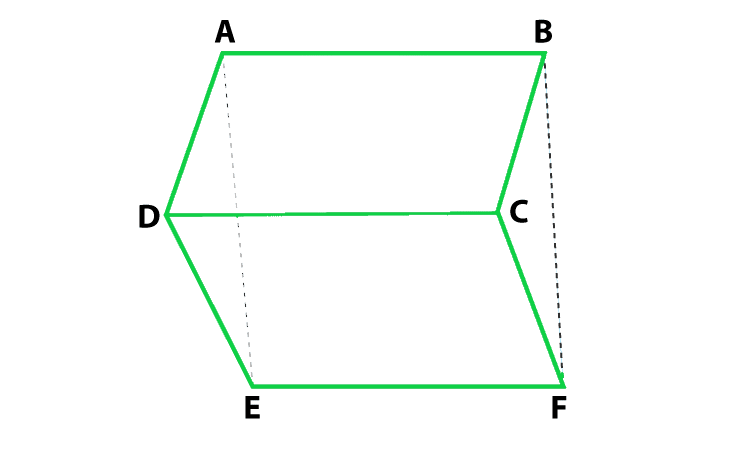Solution:

According to the question

ABCD is parallelogram, so, AD = BC

CDEF is parallelogram, so, DE = CF

ABFE is parallelogram, so, AE = BF

Proof:

DE = CF

AE = BF

So, by SSS congruence,

Hence, proved

### Question 9. Diagonals AC and BD of a quadrilateral ABCD intersect each other at P. Show that: ar(ΔAPB) × ar(ΔCPD) = ar(ΔAPD) × ar(ΔBPC).

Solution:

Construction : Draw BQ ⊥ AC and DR ⊥ AC

Prove: ar(ΔAPB) × ar(ΔCPD) = ar(ΔAPD) × ar(ΔBPC)

Proof:

Lets take L.H.S

= ar(ΔAPB) × ar(ΔCDP)

= (1/2) [(AP × BQ)] × (1/2 × PC × DR)

= (1/2 × PC × BQ) × (1/2 × AP × DR)

= ar(ΔAPD) × ar(ΔBPC)

L.H.S = R.H.S

Hence proved

### Question 10. In figure, ABC and ABD are two triangles on the base AB. If line segment CD is bisected by AB at O, show that ar(ΔABC) = ar(ΔABD).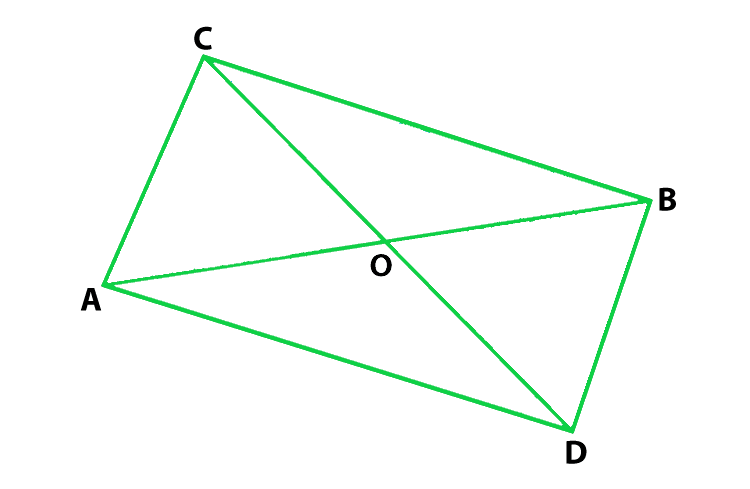Solution:

According to the question

ABC and ABD are two triangles on the base AB

and CD is bisected by AB at O,

To prove: ar(ΔABC) = ar(ΔABD).

Construction: CP ⊥ AB and DQ ⊥ AB.

Proof:

First we find the area of ΔABC = 1/2 × AB × CP         …..(i)

Now we find the area of ΔABD = 1/2 × AB × DQ              …..(i)

So, in ΔCPO and ΔDQO,

∠CPO = ∠DQO           (Each 90°)

CO = OD                    (Given)

∠COP = ∠DOQ                           (Vertically opposite angles are equal)

So, by AAS congruency

ΔCPO ≅ ΔDQO

So, by C.P.C.T

CP = DQ        …..(iii)

So, from equation (i), (ii), and (iii)

ar(ΔABC) = ar(ΔABD)

Hence proved

Attention reader! All those who say programming isn’t for kids, just haven’t met the right mentors yet. Join the  Demo Class for First Step to Coding Coursespecifically designed for students of class 8 to 12.

The students will get to learn more about the world of programming in these free classes which will definitely help them in making a wise career choice in the future.

My Personal Notes arrow_drop_up